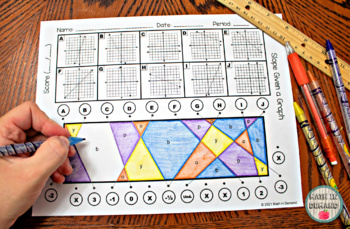DID YOU KNOW:
Seamlessly assign resources as digital activities

Learn how in 5 minutes with a tutorial resource. Try it Now

Learn More# 8th Grade Math Stained Glass GROWING BUNDLE8th
Subjects
Resource Type
Formats Included
• Zip
Pages
N/A
\$20.00
Bundle
List Price:
\$22.00
You Save:
\$2.00
\$20.00
Bundle
List Price:
\$22.00
You Save:
\$2.00

#### Products in this Bundle (6)

showing 1-5 of 6 products

### Description

This is a GROWING BUNDLE for all of my 8th Grade Math Stained Glass Activities. Currently, this bundle includes the following math concepts:

1.) Solving Two-Step Equations

2.) Solving Two-Step Equations with Fractions

3.) Solving Two-Step Equations with Parentheses

4.) Solving Equations with Variables on Both Sides

5.) Slope Given a Graph

6.) Slope Given a Table

7.) Slope Given 2 Points

8.) Slope Given Standard Form (Convert to Slope-Intercept Form)

9.) Pythagorean Theorem in the Coordinate Plane

10.) Pythagorean Theorem Given Diagram

11.) Pythagorean Theorem Given A, B, C

12.) The Converse of the Pythagorean Theorem

13.) Solving Systems of Equations by Graphing

14.) Solving Systems of Equations by Substitution

15.) Solving Systems of Equations by Elimination

16.) Solving for x using Angle Relationships (Easier)

17.) Solving for x using Angle Relationships (Harder)

18.) Solving for an Angle (Complementary, Supplementary, Vertical, & Adjacent)

19.) Volume of a Cylinder

20.) Volume of a Cone

21.) Volume of a Sphere

22.) Volume of a Cone, Cylinder, & Sphere

Coming Soon!

23.) Two-Way Tables

Don't miss out on this HUGE deal! The price increases every time I add a new Stained Glass resource.

Total Pages
N/A
Included
Teaching Duration
N/A
Report this Resource to TpT
Reported resources will be reviewed by our team. Report this resource to let us know if this resource violates TpT’s content guidelines.